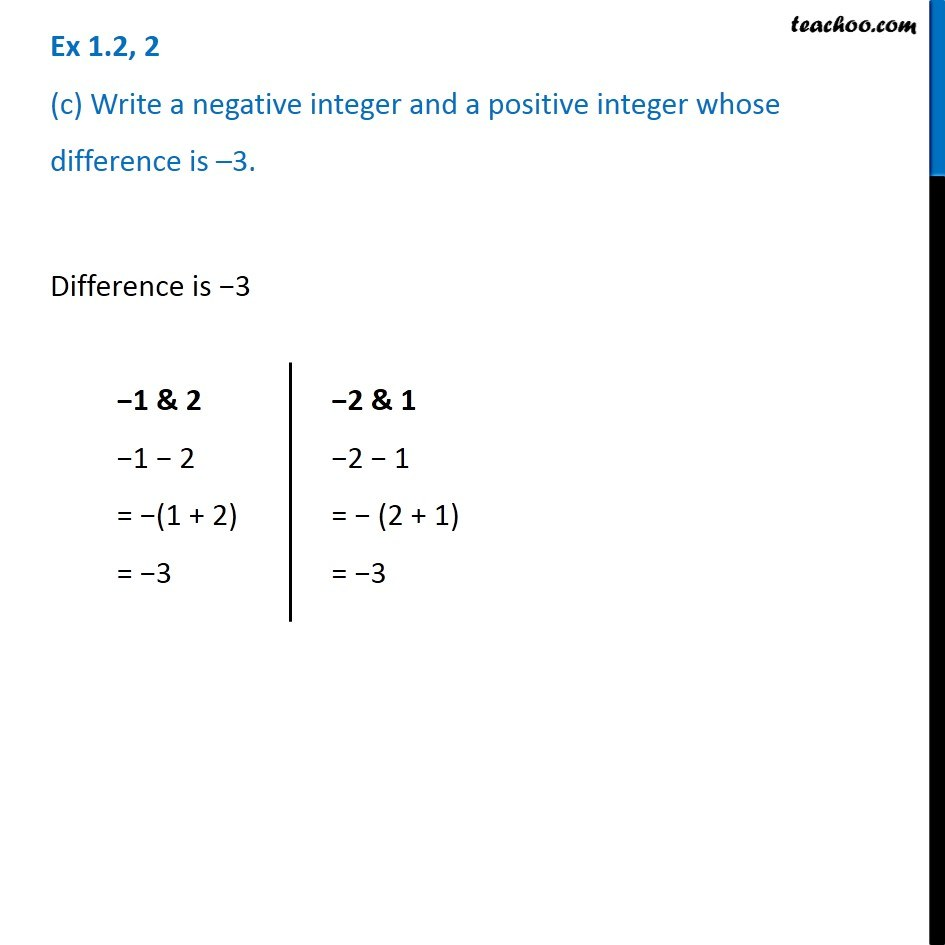1. Chapter 1 Class 7 Integers
2. Serial order wise
3. Ex 1.2

Transcript

Ex 1.2, 2 (c) Write a negative integer and a positive integer whose difference is 3. Difference is 3 1 & 2 1 2 = (1 + 2) = 3 2 & 1 2 1 = (2 + 1) = 3